## Motion Near the Earth

The earth rotates with and angular frequency of about1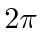radians per (sidereal) day. The axis of the earth's rotation goes through the north and south poles of the earth. The direction of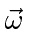goes from south to north pole. The axis is at about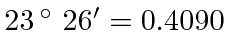radians from the plane of the earth's orbit, causing the observed seasons.

The primary effect of the centrifugal force is to change the magnitude and direction of the apparent acceleration of gravity near the earth.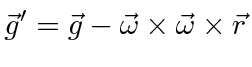Since force only depends on latitude and the radius of the earth, it is a constant correction to gravity, given a latitude. The magnitude of the centrifugal force is biggest at the equator. The average radius of the earth is 6367 km. Lets compute the magnitude ofhere.So, at the equator the apparent acceleration of gravity is.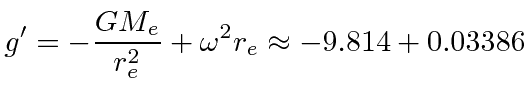It is a small but easily measurable correction to gravity.

Lets analyze the effect of the centrifugal acceleration at all latitudes, under the assumption that the earth is spherical. At non-zero latitude the first cross product, so the magnitude of the centrifugal acceleration is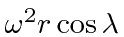and the direction is outward perpendicular to the axis of rotation. In the local coordinate system, this means that there is a radial component of the centrifugal acceleration which reduces the effectiveand there is a component pointing along a line of longitude toward the equator of magnitude. This component has very small effect on the magnitude ofbut changes its direction somewhat. Plumb bobs will hang in the direction of the effectiveso this will locally be defined as the vertical direction.

Of course if the earth were a fluid of uniform density, it would take on a shape such that the effectivewould be normal to the surface of the fluid. Newton calculated that this would make radius of the earth at the equator one part in 230 larger than the radius at the poles. The earth would take on the shape of an oblate spheroid. With much of the earth molten, it is easily deformable into this shape, however, the density is much larger at small radius than it is at the surface. Recent measurements give a somewhat smaller effect of one part in 298.25642. The oblate spheroid is the baseline for measurements of the earths shape. Gravity actually depends on the local mass density of the earth but the baseline model ofas a function of latitude is approximately.It is clear from this formula that objects will be lighter at the equator than at higher latitudes.

Besides the forces due to rotation, there are some effective forces due to the acceleration of the coordinate system. The earth is essentially in free fall in the gravitational field of the moon and the sun, so that the net force of gravity is exactly canceled by the acceleration of the earth. Only the net force is canceled. Since the effective force varies with position on the earth, there are tidal forces that cause the oceans to rise and fall.

Jim Branson 2012-10-21